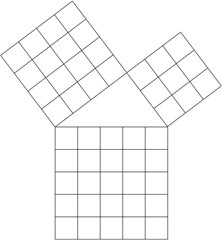### Fermat’s Last Theorem[Concrete/Interesting] A while back I saw a BBC Horizon programme about Fermat’s Last Theorem and the mathematically heroic work by Andrew Wiles in raising the Taniyama-Shimura conjecture from a mere conjecture to a theorem and using this to show a contradiction between the predictions of the epsilon conjecture and Wiles’ proof that all such elliptic curves must be modular. This contraction implies there are no solutions to Fermat’s equation, hence Fermat’s equation is true.

Like many, I was excited about this proof and although difficult to follow (Elliptic Curves are hard to understand, no kidding), the proof was without a doubt a clever piece of late 20th century mathematical wizardry, full of deep insight and imagination.

But something was wrong. It was all to clever, all too complex, all to contemporary. So to mark the birth of my new baby boy, Khaliq, I decided to provide a simple proof, maybe not complete in mathematical rigor and probably nowhere near robust enough for Cambridge Mathematics, possibly not even correct, but something I think 17th century mathematicians would have found interesting. See what you think.To my son, Khaliq George Mahmood, born 26th May at 11.24pm (7lb, 12oz). Hope you find life as fascinating as I have.Fermat’s conjecture is so tidy, so elegant and simply described as:That is to say, for all integers, except 2, the equation has no non-zero integer solutions. For 2, where we square x and y, the sum of the squares of x and y is equal to the square of z

For n = 2, this may be familiar to some of you, and is known as The theorem of Pythagoras. Pythagoras, mathematician, philosopher and scientist, provided a proof for his equation based on logic and simple geometry. In fact, the equation was known to many at the time, but is was the application of mathematical rigor that gave Pythagoras the title over the equation.

It is from the relationship between the equation and a right angle triangle that I will start my ‘proof’’.

### Mathematical Rigor and Proof

Mathematics is complicated, at least for the majority of us.I don’t want to pretend I am a mathematician or blessed with anything but a cursory level of competence in mathematics.

For a proof to stand on it’s own two legs it must be a convincing argument and this must be based on deductive reasoning which shows a statement to be true in all cases. Ok, so I don’t think I’m going to be able to do that. But I hope I can present an somewhat unproven proposition that I believe to be true – that, at least is a conjecture, and is something others may be able to prove.

So away with the shackles of mathematical rigor, eyes down for what I hope is an interesting conjecture:

### Not Now Nigel’s Conjecture

Above n=2 where n is the geometric dimension, there are no simple object with 3 ‘flat’ boundaries.

By way of explanation, for n=2, the equation maps to a 2 dimensional polygon with three flat or straight line boundaries, commonly known as a Triangle:But for higher dimensions, there are no object with 3 ‘flat’ sides. For example, for 3 dimensions, there is no polyhedron with 3 planar surfaces. The nearest is something like a Tetrahedron with 4 sides.

In proving this conjecture and in saying that Fermat’s equation is represented by this geometry, there lies a simple proof to Fermat’s Last Theorem. Q.E.D.